# Introduction to Statistics for Research: Standard error and confidence intervals

## Preliminary: the Normal distribution

Many statistical methods are only valid if we can assume that our data follow a distribution of a particular type, called the Normal distribution. Many naturally occurring biological variables follow distributions which are very similar to the Normal.

For example, Figure 1 shows the distribution of birthweight in 1603 singleton term births (37 weeks gestation or more) to Caucasian mothers at St Georges Hospital, London, with the curve which represents the Normal distribution.

Figure 1. Histogram of birth weight of term births with corresponding Normal distribution curve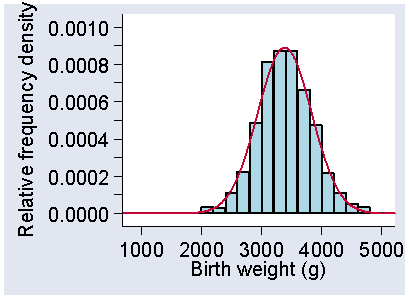d

Figure 2 shows a Normal distribution superimposed on the distribution of height in women with venous ulcers.

Figure 2. Histogram of heights of women with venous ulcers, with corresponding Normal distribution curve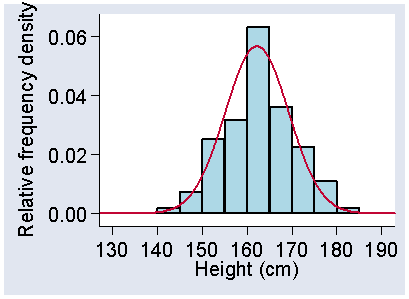d

In both Figure 1 and Figure 2, the histogram representing the distribution and the smooth curve representing the Normal distribution appear to be quite close. It seems quite plausible that the data follow the distribution described by the Normal curve.

The curves in Figure 1 and Figure 2 have similar shapes, but they are not identical. In Figure 1 the middle of the curve is at 3384 g and in Figure 2 the middle of the curve is at 162.2 cm, for example. The Normal distribution is not just one distribution, but a family of distributions. The particular member of the family that we have is defined by two numbers, called parameters. Parameter is a mathematical term meaning a number which defines a member of a class of things. It is a much-abused word. The parameters of a Normal distribution happen to be equal to its mean and variance. To obtain Figure 1, we calculated the mean birthweight, 3384 g, and standard deviation, 449 g, or the variance 201601 g. The Normal distribution equation gives us the relative frequency density for each value of birthweight, which we plot on the graph.

The Normal distribution is important for two reasons. First, many natural variables follow it quite closely, certainly sufficiently closely for us to use statistical methods which require this. Second, even when we have a variable which does not follow a Normal distribution, if we the take the means of several samples of observations, such means will follow a Normal distribution.

The Normal distribution has many useful properties, but one which is not helpful: there is no simple formula linking the variable and the area under the curve. Hence we cannot find a formula to calculate the frequency between two chosen values of the variable, nor the value which would be exceeded for a given proportion of observations. Mathematicians love a challenge, and they developed several numerical methods for calculating these things with acceptable accuracy. These were used to produce extensive tables of the Normal distribution. Examples can be found in many statistics books and specialised books of tables. Now that computers are everywhere and statistical software is readily available and easy to use, these tables have become rather redundant and I have not included them in these notes. The numerical methods for calculating Normal frequencies have been built into statistical computer programs and computers can estimate them whenever they are needed.

Two numbers from these tables are worth giving, however. We expect 68% of observations to lie within one standard deviation from the mean, and 95% to lie within 1.96 standard deviations from the mean. This is true for all Normal distributions, whatever the mean, variance, and standard deviation. Compare these figures of 68% within one SD of the mean and 95% within 1.96 SD to the about 2/3 (67%) within one SD and about 95% within 2 SD given above in the previous lecture. (Of course, 1.96 is almost equal to 2.) Hence, if we can assume that our observations follow a Normal distribution, we can estimate the 95% range from the mean minus 1.96 standard deviations to the mean plus 1.96 standard deviations. This will vary much less from sample to sample that will the 95% range estimated directly from the centiles.

## Sampling

The work of health care professionals is usually focussed on individual patients. It is the individual they seek to help and the individual in whom they are interested. Research is different, because in health care research we obtain information from our research subjects to enable us to find out something more general, which would apply to a wider group of people. For example, if we have a group of patients in a clinical trial, from the research point of view we are not interested in them as individuals or as patients. We are interested in what they can tell us about a wider group of patients, those having the same disease who will present in the future, both in our own location and in other parts of the country and other parts of the world. Most research data come from subjects we think of as samples drawn from a larger population. In this lecture we look at what a sample can tell us about that population.

The notion of sampling is familiar in health care. If we want to know the blood glucose level of a person with diabetes, we do not take all the patients blood to determine how much glucose is in it. We take a small sample obtained from a pinprick. We use the concentration of glucose in this sample to give us an estimate for the blood in the entire body. In the same way, if we wish to know by how much more elastic multilayer high compression bandaging improves healing of venous ulcers compared with inelastic multilayer compression bandaging, we do not include all the patients in the world. We use a sample of patients and use the difference between the two types of bandage found in them to provide an estimate for patients in general.

The problem is that not all samples produce the same estimate. If we take a blood sample to estimate blood glucose, then repeat it with a second sample, we do not necessarily get the same measurement. Three successive measurements from my fingers, made in quick succession, were 6.0, 5.9, and 5.8. Which of these is correct? The answer is that none of them are; they are all estimates of the same quantity, but we do not know whether any of them is exactly right.

In the same way, three trials of elastic versus inelastic multilayer compression bandaging for venous ulcers produced the following differences in the percentage of patients achieving complete healing: 13, 25, and 20 percentage points in favour of elastic bandaging (Fletcher et al., 1997). These are all estimates of the advantage to elastic bandaging. We would not expect them all to be the same, because of the natural random variation between samples. (In this case there are other differences, too, because there are differences between the three trials in time of follow-up and details of the treatment.) The estimates which we might obtain from all the possible samples drawn in the same way as ours have a distribution. We call this the sampling distribution.

## Sampling distributions

To see how sampling works, we shall first look at an example where is it easy to find the exact answer because we know it in advance. This is a trick statisticians use to check their methods. We shall look at the problem of estimating the mean of a measurement from a sample and see what happens as we increase the size of the sample. We shall use as our example an ordinary, six-sided die.

Rolls of the die will produce a score that will act as the original measurements that we make. Rolling a die can produce one of six numbers: 1, 2, 3, 4, 5, or 6. Each of these will happen in the same proportion of rolls of the die, 1/6. (Experience with board games suggests that this is not true, but, theoretically, it is.) The first panel of Figure 3 shows the proportions of rolls which would give each possible score, all equal to 1/6 or 0.167.

Figure 3. Distributions for the score from rolling a single die, the mean of two dice scores, the mean of four dice scores, and the mean of six dice scores.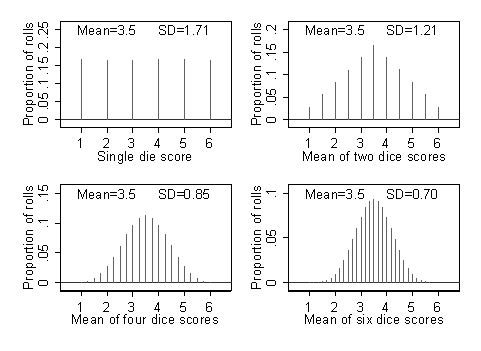d

This is the distribution of scores for a single roll of a die. In Figure 3, the points are shown as vertical lines rising from zero, to emphasise that only a few discrete values are possible.

In this example, of course, we know that the true or population mean is 3.5. It is the average of 1, 2, 3, 4, 5, and 6. Hence the average of all the possible rolls will be (1+2+3+4+5+6)/6 = 3.5. This is the mean of this distribution, the average score we would expect to get over very many rolls of the die. We can also work out the standard deviation of the scores we would get, just as we worked out the standard deviation of a sample in the last lecture. This is 1.71, and is the standard deviation of the distribution.

Now let us put ourselves in the position of not knowing what the average score would be. We take a sample of dice rolls to enable us to estimate the mean. We might roll two dice and calculate the mean (or average) of the two scores to provide the estimate of the mean for the population of all dice rolls. We can find the distribution for the mean of two dice scores quite easily. The first die can show six different faces, and for each of these the second die can show six different faces, so there are 6?6 = 36 possible outcomes altogether. The lowest possible value of the mean score is 1.0, but this cannot happen often. Both dice would have to show a one, so we only get a mean equal to 1.0 once in 36 rolls, the proportion of rolls when it would occur is 1/36 = 0.028. The next possible mean is 1.5, when one die shows a score of one and the other a score of two. This can happen twice in 36 rolls, as the first dice can show one and the second two, or the first two and the second one. The proportion of rolls when a mean equal to 1.5 would occur is 2/36 = 0.056. We can list all the possibilities for the two dice and calculate their means, and hence find the proportion of rolls on which each possible mean can occur. These are shown in the second panel of Figure 3. We can also calculate the mean and standard deviation of these averages of two dice scores. The mean is 3.5, as before, but the standard deviation is not the same. It is 1.21, which is less than the 1.71 for a single die.

As an estimate of the mean of the population of possible die scores, rolling a single die is not going to be much use. The results would be tremendously variable. Rolling two dice and using the mean of the two scores would be a bit better. The estimates would be more likely to lie near the middle of the distribution of possible values than at the ends and hence would be closer to the true mean. The more dice we roll, the more accurate we might expect our sample mean to be as an estimate for the population mean. Figure 3 shows what happens when we roll four or six dice. These would give us the sampling distributions for the mean of four dice scores and for the mean of six dice scores.

There are several things to notice in Figure 3. First, the mean of the distribution is always the same, 3.5. When we take several observations and average them, the mean of many such averages is the same as the mean for the distribution of single observations. Second, the distributions become less variable as the number of dice increases. There are much smaller proportions of rolls producing means close to 1.0 or 6.0 and the standard deviations get smaller. Third, the shape of the distribution changes as the number of dice increases. The shape it tends towards as the number of dice increases may look familiar. It is similar to the Normal distribution curve. Figure 4 shows the distributions with superimposed the curve for the member of the Normal distribution family which has the same mean and standard deviation as the dice roll distribution.

Figure 4. Distributions for the score from rolling a single die, the mean of two dice scores, the mean of four dice scores, and the mean of six dice scores, with a Normal distribution curve.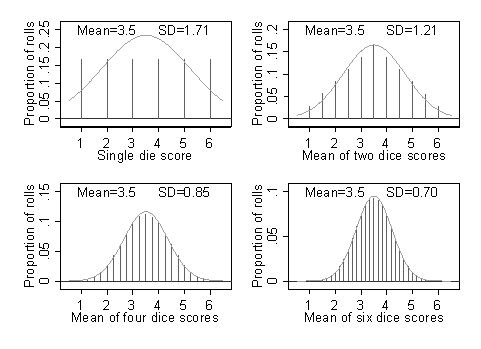d

For one die, the Normal distribution curve is nothing like the proportions for the six possible scores. For two dice, the Normal is closer, but not a good fit as the Normal would go on below 1.0 and above 6.0, indicating possible means there, which we could not have. For six dice rolls the Normal distribution curve is a pretty good fit.

These three things are quite general and will happen with almost any observations we care to make, whether they are dice rolls or measurements of blood pressure. If we take a sample of several observations and find their mean, whatever the distribution of the original variable was like, such sample means will have a distribution which has the same mean as the whole population. These sample means will have a smaller standard deviation than the whole population, and the bigger we make the sample the smaller the standard deviations of the sample means will be. Finally, the shape of the distribution gets closer to a Normal distribution as the number in the sample increases.

We call any number we calculate from a sample, such as a mean, proportion, median, or standard deviation a statistic. Any statistic which is calculated from a sample will have a sampling distribution. A lot of statistical theory is about working out what the correct sampling distribution is for different statistics. We are going to take that as read, and assume that, whatever the problem, the theorists have got it right. These sampling distributions are built into the statistical methods which have been developed. For example, in t tests the sampling distribution used is Students t distribution. Analyses using t tests are reported with results obtained using this distribution. The person doing or reporting the analysis does not need to know anything about the t distribution to carry out the analysis or to understand its implications, nor does the reader of the report.

## Standard errors

Standard error is one of the things which we can use to describe how good our estimate is. We often see estimates reported with a standard error attached. Statistical computer programs print out standard errors by the hundred. The standard error comes from the sampling distribution. The standard deviation of the sampling distribution tells us how good our sample statistic is as an estimate of the population value. We call this standard deviation the standard error of the estimate. Hence the standard error of the mean of six dice scores is 0.70, as shown in Figure 3.

People find the terms standard error and standard deviation confusing. This is not surprising, as a standard error is a type of standard deviation. We use the term standard deviation when we are talking about distributions, either of a sample or a population. We use the term standard error when we are talking about an estimate found from a sample.

In the dice example, we know exactly what the distribution of the original variable is because it comes from a very simple randomising device (the die). In most practical situations, we do not know this. If we think about the elastic vs. inelastic bandages, for example, the first trial gave us the difference between the two percentages whose ulcers were completely healed to be 13. This is an estimate of the difference in the population of all venous ulcer patients, but what is its standard error? There were 31 healed out of 49 patients in the elastic bandage group and 26 out of 52 in the inelastic bandage group (Northeast et al., 1990, cited in Fletcher et al., 1997). On theoretical grounds, we know the family of distributions which the difference will be from. In this case, it is approximately Normal. Which member of the Normal distribution family we have depends on the proportion of the whole patient population who would heal if given elastic bandages and the proportion of the whole patient population who would heal if given inelastic bandages. We do not know these and there is no way that we could know them. However, we can estimate them from the data, using the sample percentages 63% (31/49) and 50% (26/52). We then calculate what the standard error would be if the unknown population percentages were, in fact, equal to these sample percentages. This estimated standard error can then be used to assess the precision or the estimate of the difference.

Now things get very confusing, because we call this estimated standard error the standard error also. For our difference between the two percentages with healed ulcers, which was 13 (63-50), the standard error is 10 percentage points. (I omit the technical details of how this figure was arrived at.)

Standard errors are often published in research papers. The standard error of an estimate tells us how variable estimates would be if obtained from other samples drawn in the same way as one being described. Even more often, research papers include confidence intervals (below) and P values (next lecture) derived using them.

Estimated standard errors can be found for many of the statistics we want to calculate from data and use to estimate things about the population from which the sample is drawn. The mathematical formulae which are used to find them are built into the statistical computer programs which are used to calculate these statistics, so researchers analysing data do not need to remember them, or even to understand them. If the researcher doesnt need to know them, the reader doesnt either. This is my justification for omitting them from these notes, but if you want to know more you will find them, including one for the difference between two proportions, in Bland (2000).

## Confidence intervals

Confidence intervals are another way to think about the closeness of estimates from samples to the quantity we wish to estimate. Some, but not all, confidence intervals are calculated from standard errors. Confidence intervals are called interval estimates, because we estimate a lower and an upper limit which we hope will contain the true values. In mathematics, the numbers defined by being above a lower limit and below an upper one is called an interval, hence an interval estimate is an estimate in the form of a continuous range of possible values. An estimate which is a single number, such as the difference we observed from the trial, is called a point estimate.

It is not possible to calculate useful interval estimates which always contain the unknown population value. There is always a very small probability that a sample will be very extreme and contain a lot of either very small or very large observations, or have two groups which differ greatly before treatment is applied. So we calculate our interval so that most of the intervals we calculate will contain the population value we want to estimate. Often we calculate a confidence interval: a range of values calculated from a sample so that a given proportion of intervals thus calculated from such samples would contain the true population value. For example, a 95% confidence interval calculated so that 95% of intervals thus calculated from such samples would contain the true population value. This definition is very hard for most of us to get our heads round, so I shall try to show how it works.

For example, for the venous ulcer bandage study we have an estimated difference of 13 and a standard error of 10. The sampling distribution is approximately Normal, with mean equal to the unknown population difference and standard deviation equal to the standard error, estimated to be 10. We know that 95% of observations from a Normal distribution are closer than 1.96 standard deviations to the mean. Hence 95% of possible samples will have the difference closer to the unknown population mean than 1.96?10 percentage points. If we estimate our unknown population value to be between the observed sample value minus 1.96 standard errors and the observed sample value plus 1.96 standard errors, that range of values would include the population value for 95% of possible samples. Thus the 95% confidence interval is 13  1.96 × 10 = 7 to 13 + 1.96 × 10 = 33 percentage points. Hence we estimate that the true difference in the population lies between 7 and +33 percentage points. What about the estimates from the other samples? The second estimate was 25 percentage points (Callam et al., 1992, cited in Fletcher et al., 1997) and the 95% confidence interval is +9 to +42. The third estimate was 20 percentage points (Gould et al., unpublished, cited in Fletcher et al., 1997) and the 95% confidence interval is 10 to +50.

Figure 5 shows the confidence intervals for the three studies.

Figure 5. Confidence intervals for three trials comparing elastic and inelastic bandages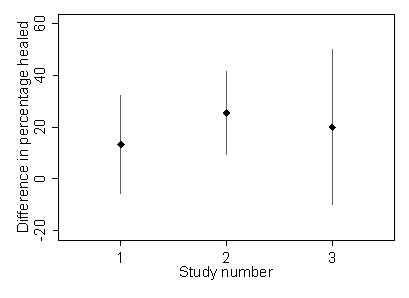d

(This type of plot, with a series of estimates and their confidence intervals, is called a forest plot, because the vertical lines resemble trees.) The width of the confidence interval depends on how many observations there were and the third study was smaller than the others. These confidence intervals all overlap, so they are quite consistent with the same unknown true value, which could lie within all of them.

Confidence intervals do not always include the population value. If 95% of 95% confidence intervals include it, it follows that 5% must exclude it. In practice, we cannot tell whether our confidence interval is one of the 95% or the 5%. Figure 6 shows a computer simulation of a trial of elastic bandaging.

Figure 6. Simulation showing 100 trials comparing groups of size 49 and 53, with proportions healing set to 57% in the elastic bandage group and 37% in the inelastic bandage group.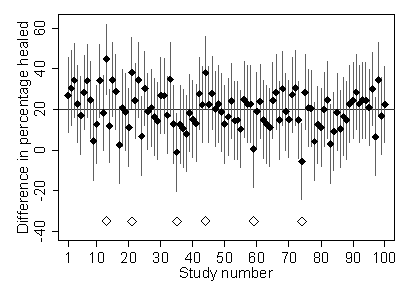d
Large open diamonds indicate confidence intervals which do not include the population difference, 20 percentage points.

I have used the sample sizes in the Eastwood trial, 49 and 52 patients in the elastic and inelastic bandage groups, and assumed that in whole population of patients, the proportion of patients experiencing total healing would be 57% in the elastic bandage group and 37% in the inelastic bandage group. (These are the proportions obtained by simply combining the three trials in Figure 5.) In Figure 6, of the 100 trials six 95% confidence intervals do not include the population difference, 20 percentage points. If we kept on simulating trials, eventually we would expect to see 5% of the intervals having the population value outside the interval, but in any particular simulation of 100 trials we might not get exactly 5%. Here, we have 6%, which is close enough.

## Examples of confidence intervals

Table 1 shows serum cholesterol measured on a sample of 86 stroke patients.

We have previously looked at the mean, median and standard deviation of these data. We can find confidence intervals for these as estimates of the same quantities for the population from which the sample was drawn.

The sample mean is 6.34 mmol/L, the point estimate, and the 95% confidence interval is 6.04 to 6.64. This was calculated using the standard error.

The median is 6.15 mmol/L and a 95% confidence interval is 5.7 to 6.5. This was calculated by a method which used the Binomial distribution, rather than using a standard error. Note that mean is better estimated than median, its confidence interval being narrower.

The standard deviation is 1.40. It, too, has a 95% confidence interval. Using the standard error method, we get 1.19 to 1.61 mmol/L. There is another method, slightly different, called the chi-squared method. This also gives 1.19 to 1.61 mmol/L. However, if we quote more decimal places, they are not quite the same. The standard error method gives 1.1894 to 1.6102 mmol/L and the chi-squared method 1.1896 to 1.6096 mmol//L. Note that there may be more than one way to calculate a confidence interval and they may not give exactly the same answer.

## Do we always use 95% confidence intervals?

So far we have looked at 95% confidence intervals, chosen so that 95% of intervals will include the population value. The choice of 95% is just that, a choice. There is no reason why we have to use it. We could use some other percentage, such as 99% or 90% confidence intervals. As you might expect, if 99% of intervals include the population value, they must be wider than 95% confidence intervals. 90% intervals are narrower. Figure 7 shows the simulation of Figure 6, with 95%, 99%, 90% and 50% intervals.

Figure 7. Simulation showing 100 trials comparing groups of size 49 and 53, with proportions healing set to 57% in the elastic bandage group and 37% in the inelastic bandage group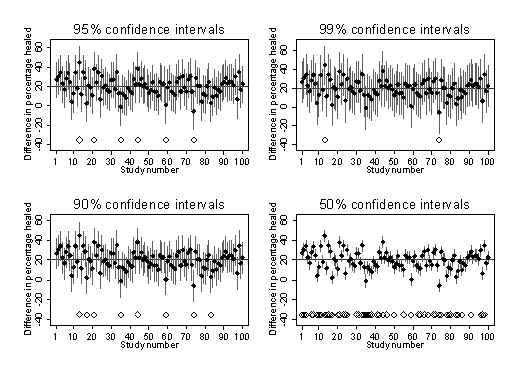d
Large open diamonds indicate confidence intervals which do not include the population difference, 20 percentage points.

The 99% confidence intervals are wider and in this particular simulation 98 intervals include the population value, more than the 94 of the 95% confidence intervals which include it and less than the 92 of the 90% confidence intervals which do so in this particular simulation. The 99% confidence interval is 19% wider than the 95% confidence interval, but more intervals are correct, in that the interval estimate includes the population value. We do not gain a lot by widening the interval, that extra 19% of width includes the population value in only 4% (99%  95%) of 99% confidence intervals. On the other hand, the 90% confidence interval is 16% narrower than the 95% confidence interval, but fewer intervals are correct. The choice of 95% is a compromise between the desire to have a confidence interval which includes the population value and one which is narrow enough to provide some useful information. Occasionally researchers may want a different compromise and use 90% or 99% intervals, often without giving any indication in their reports as to why they did this.

## References

Bland M. (2000) An Introduction to Medical Statistics. Oxford University Press.

Callam MJ, Harper DR, Dale JJ, Brown D, Gibson B, Prescott RJ, Ruckley CV. (1992) Lothian Forth Valley leg ulcer healing trialpart 1: elastic versus non-elastic bandaging in the treatment of chronic leg ulceration. Phlebology 7, 136-41.

Fletcher A, Nicky Cullum N, Sheldon TA. (1997) A systematic review of compression treatment for venous leg ulcers. British Medical Journal 315, 576-580.

Newcombe, R.G. (1992) Confidence intervals: enlightening or mystifying. British Medical Journal 304, 381-2.

Northeast ADR, Layer GT, Wilson NM, Browse NL, Burnand KG. (1990) Increased compression expedites venous ulcer healing. Royal Society of Medicine Venous Forum. London: Royal Society of Medicine.

Turnbull, P.J., Stimson, G.V., and Dolan, K.A. (1992) Prevalence of HIV infection among ex-prisoners. British Medical Journal 304, 90-1.